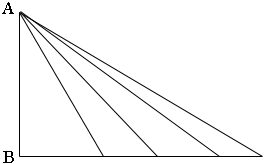Quandaries and Queries I am trying to find the hypotenuse of a right triangle with only the length of the opposite side. What is the formula as I don't have the length of the adjacent side? Can I compute it without knowing what the other two angles are? Shannon Hi Shannon, You need to know more than the length of one side to find the length of the hypotenuse in a right triangle. In the diagram below each of the triangles has the "opposite side" AB and yet the hypotenuses are of different lengths.Penny Go to Math Central